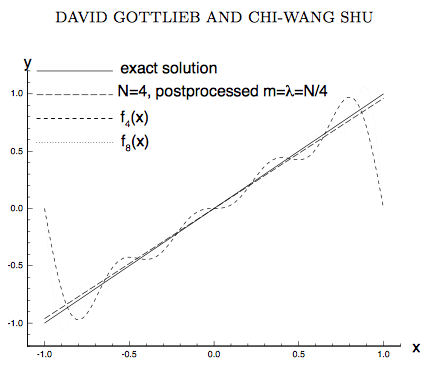# What is the importance of convergence of variation of Fourier reconstruction to that of variation of the function?

Let $f$ be a periodic function of bounded variation which jumps at a point $x_0\in\mathbb{R}$. Let $S_{N}[f]$ denote the partial Fourier sum of $f$ and let $C_{N}[f]$ denote the Cesaro partial sum. It is known that, given an interval $(a,b)$,(Also assume throughout entire question, that $f$ does not jump at either $a$ or $b$) and if $x_0\notin(a,b)$, then $\lim_{N \to \infty} V_a^bS_{N}[f] = V_a^b f$.

But when $x_0\in (a,b)$, $\lim_{N \to \infty} V_a^bS_{N}[f] = \infty$, although this is some what different for Cesaro summation where the limit exists but not equal to that of the function $f$. $\lim_{N \to \infty} V_a^b C_{N}[f]$ is finite but not equal to $V_a^b f$.

Let any general mechanism of Fourier reconstruction using a finite number (first $N$) of Fourier coefficients (like what is done by partial sum and Cesaro partial sum) be denoted as $G_N[f]$, we know it is useful if $G_N[f] \to f$ pointwise as $N\to \infty$, Fourier partial sum and Cesaro sum being two examples which obey this. My question is how important it is for a general mechanism $G_N[f]$ to not only converge pointwise to $f$ as $N\to \infty$, but also $$\lim_{N \to \infty} V_a^bG_{N}[f] = V_a^bf$$ even when $f$ jumps atleast once in $(a,b)$.

Mathematically how important it is to search for such a Fourier reconstruction mechanism? Is it anywhere given in literature, the importance of such a thing?

PS : $V_a^bf$ denoted the variation of the function $f$ in the interval $(a,b)$. Also assume throughout entire question, that $f$ does not jump at either $a$ or $b$.

You ask for a Fourier reconstruction mechanism that avoids the Gibbs phenomenon. One strategy in this direction was developed by David Gottlieb and collaborators, in a series of papers entitled ￼"On the Gibbs phenomenon: Recovering exponential accuracy from the Fourier partial sum of a nonperiodic analytic function" (part I, part II, part III, part IV, part V). A summary of this body of work was given in On the Gibbs phenomenon and its resolution (1997), and more recently in A review of David Gottlieb’s work on the resolution of the Gibbs phenomenon (2011).

The partial Fourier sum of order $$N$$ is post-processed by converting it in a series of Gegenbauer polynomials $$C_n^\lambda(x)$$ ($$n=1,2,\ldots N$$, with $$\lambda=N/4$$). The requirement for an exponentially accurate solution is that the location of the jump discontinuity is known in advance, and that the function is analytic elsewhere. The overall conclusion is that global expansions which are contaminated by local discontinuities still retain within them high order information, and the Gibbs phenomenon can be removed by post-processing.

The Gibbs phenomenon deals with the issue of recovering point values of a function from a finite set of Fourier expansion coefficients. We show that the knowledge of the expansion coefficients is sufficient for obtaining the point values of a piecewise smooth function, with the same order of accuracy as in the smooth case. This is done by using the finite Fourier expansion series to construct a different, rapidly convergent, approximation in terms of Gegenbauer polynomials. Thus the retrieval of the information should be done in a different basis than the storage of the information. The Fourier coefficients of a function contain enough information such that a different expansion, which is highly accurate, can be reconstructed.## EDIT in response to comments by the OP

For an application of this method to image processing, see Improving tissue segmentation of human brain MRI through preprocessing by the Gegenbauer reconstruction method (2003). In that application the jumps are due to segmentation of the magnetic resonance image, so their location is known in advance.

What if the location of the jumps is not known? Is there a way to locate the edges of the interval of analyticity using only knowledge of a partial set of Fourier coefficients? This problem has been addressed by Gelb and Tadmore in Detection of Edges in Spectral Data, part I, part II, part III. The method of edge detection followed by Gegenbauer reconstruction has been tested in this paper, for both one-dimensional and two-dimensional Fourier coefficients.

• "The requirement for an exponentially accurate solution is that the location of the jump discontinuity is known in advance", Nothing is known in advance! One can never get the exact location of jump from finite Fourier coefficients. Jul 22, 2015 at 4:26
• Wouldn't that depend on the type of application? In the particular application (to segmented MRI scans) I mention above the jumps are at known locations. I actually thought the question of the OP was about Fourier reconstruction methods in general, not tied to some particular application. Have I misunderstood the question? Jul 22, 2015 at 7:56
• You got the question almost right, but my the intention was not for any particular application, it is asking for a theoretical result and not tied to any application. I must say your answer is useful in many ways, but the intention of the question is to find a theoretical result for any function without laying out any physical or application oriented constraints like knowledge of jumps, etc. Jul 22, 2015 at 10:08
• Here in OP, Fourier reconstruction does not confine to using only finite Fourier coefficients but using all coefficients in the form of $N\to\infty$, asking for an asymptotic result, just like convergnce of Fourier series. Fourier series itself is one type of Fourier reconstruction. Jul 22, 2015 at 10:12
• Basically I dont want any conditions on $f$ like knowledge of jumps. Jul 22, 2015 at 10:14